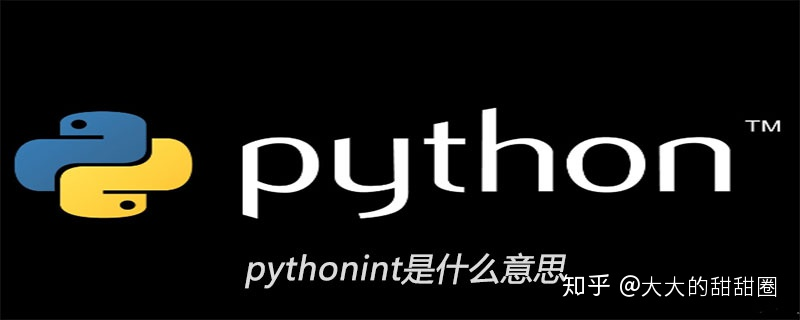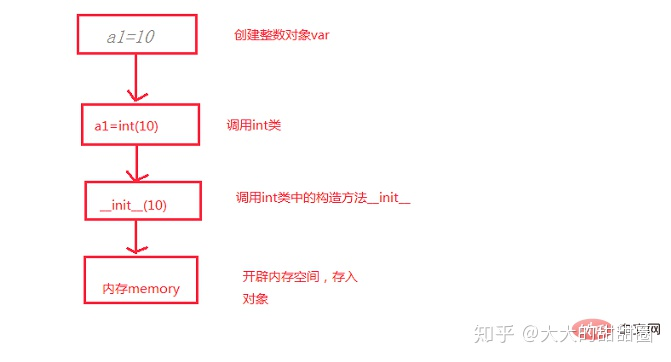• 学习python的同学可能会有这样的问题，为什么python中会存在int(10)这个语句，既然a=10和a=int(10)效果相同，那么就不需要存在int(10)了呗，那么让我们来看看下面的图片 ...学习python的同学可能会有这样的问题，为什么python中会存在int(10)这个语句，既然a=10和a=int(10)效果相同，那么就不需要存在int(10)了呗，那么让我们来看看下面的图片由图可以看到，我们 在代码中写入a=10,其实计算机最终还是要调用int的构造方法，也就是说a=10,最终在计算机中还是变成a=int(10)，不明白的话，那么来看看int的内部构造函数原型def init(self,x,base):中有三个参数，第一个参数self：指的是当前对象，x: 指控制台输入的数据，base：表示当前这个数是几进制，如果不写，默认base=10进制，例如：int(‘1010001’,2)这句代码解析为：x=1010001,base=2
最终代码会转化为10形式输出。
如果大家如果在学习中遇到困难，想找一个Python学习交流环境，可以加入我们的Python学习圈，点击我加入吧，会节约很多时间，减少很多遇到的难题。以上就是python中int是什么意思的详细内容

展开全文• ## Python中int 用法详解

万次阅读 多人点赞 2019-05-02 21:29:33
Python int 的少见用法之进制转换 文章目录Python int 的少见用法之进制转换0. 参考资料1. int 的常见用法2. int 用于进制转换 0. 参考资料 Python 官方文档 1. int 的常见用法 众所周知，int ...
Python 中 int 用法详解
欢迎转载，转载请注明出处！

文章目录
Python 中 int 用法详解0. 参考资料1. int 的无参数调用2. int 接收数字作为参数3. int 接收字符串作为参数：类型转换4. int 用于进制转换5. 版本特性

0. 参考资料
Python 官方文档

1. int 的无参数调用
不带参数直接 int()，得到的是整数 0。
num = int()
print(type(num))
print(num)
"""
<class 'int'>
0
"""


2. int 接收数字作为参数
int 接收整数作为参数，其实没什么好说的，还是得到原来的那个整数。int 接收浮点数作为参数，会截取该浮点数的整数部分，返回截取后的整数。
print(int(2.9))    # 2
print(int(2.1))    # 2
print(int(-2.9))   # -2
print(int(-2.1))   # -2
print(int(3.0))    # 3


注意：int 没有四舍五入的功能，它做的事情是 截断，截断，截断！

3. int 接收字符串作为参数：类型转换
众所周知，int 函数可以将一个字符串转换为整数，这也是它最常见的用法：
s = input('请输入一个整数：')
print(type(s))
"""
<class 'str'>
"""

num = int(s)
print(type(num))
"""
<class 'int'>
"""


注意：确切来讲 int 是一个类，而不是函数，虽然它的首字母没有大写。

假如字符串 s 是一个浮点数形式的字符串，比如 '-3.14'，直接用 int 进行类型转换会报 ValueError 错，如下所示：
s = '-3.14'
num = int(s)
"""
ValueError: invalid literal for int() with base 10: '-3.14'
"""

如果想要把浮点数形式的字符串转换为整数，需要先使用 float 把字符串转换为浮点数，再对该浮点数使用 int，把浮点数截取为整数，如下所示：
s = '-3.14'
num = int(float(s))
print(f'num = {num}')
"""
num = -3
"""


4. int 用于进制转换
到目前为止介绍的都是 int 的常见用法，接下来介绍的是相对少见的用法，int 可以将 2 进制到 36 进制的字符串、字节串（bytes）或者字节数组（bytearray）实例转换成对应的 10 进制整数。具体的调用形式为：int(x, base=10)，其中 x 即为字符串、字节串或字节数组的实例。
参数说明
参数 x 必须是合法的整数字面量 integer literal。 整数字面量前面可以有正负号，但是正负号与数字之间不能有空格。 整数字面量两端可以出现任意数量的空白，包括空格键、制表符、换行符等等。 a-z 或者 A-Z 分别表示 10-35 的数字。 二进制数字可以用 0b 或者 0B 做前缀，八进制数字可以用 0o 或者 0O 做前缀，十六进制数字可以用 0x 或者 0X 做前缀，前缀是可选的。base 默认是 10，还可以取 0 以及 2 到 36，不能是 1，因为没有 1 进制。 base 为 0 的时候会按照代码字面量（code literal）解析，即只能把 2、8、10、16 进制数字表示转换为 10 进制。对于 2、8、16 进制必须指明相应进制的前缀，否则会按照 10 进制解析。
默认情况：base=10
x = '6'
num1 = int(x)
num2 = int(x, 10)
print(num1)
print(num2)
"""
6
6
"""

其他进制转为 10 进制
x = '10'
num1 = int(x, 2)
num2 = int(x, 8)
num3 = int(x, 16)
print(num1)
print(num2)
print(num3)
"""
2
8
16
"""

x = 'z'
num3 = int(x, 36)
print(num3)
"""
35
"""

带正负号、空格的整数字面量
# 带正号
x = '+a0'
num = int(x, 16)
print(num)
"""
160
"""

# 带负号
x = '-a0'
num = int(x, 16)
print(num)
"""
-160
"""

# 两端带空白
x = '  \t  +a0\r\n  '
num = int(x, 16)
print(num)
"""
160
"""

base=0 时按照代码字面量直接解析
x = '10'
num = int(x, 0)
print(num)
"""
10
"""

x = '0b10'
num = int(x, 0)
print(num)
"""
2
"""

x = '0o10'
num = int(x, 0)
print(num)
"""
8
"""

x = '0x10'
num = int(x, 0)
print(num)
"""
16
"""


5. 版本特性
3.4 版本变化
如果 base 参数不是 int 类的实例，那么会调用它的 base.__index__ 方法，该方法返回一个整数供 base 参数使用。之前的版本调用的是 base.__int__ 而不是 base.__index__。
3.6 版本变化
代码字面量（code literal）中允许使用下划线 _ 来对数字进行分组。
3.7 版本变化
x 现在变成了强制位置参数 positional-only parameter。

注意：也就是说 x 不能通过 x=... 这种关键字参数形式来指定，以下是我在 Python 3.6 当中的试验，可见 3.6 版本还是可以的。然而 Python 3.7 版本会报错！

# Python 3.6
x = 'a'
num = int(x=x, base=16)
print(num)
"""
10
"""
# 调过来也可以
x = 'a'
num = int(base=16, x=x)
print(num)
"""
10
"""

# Python 3.7 会报错
>>> x = 'a'
>>> num = int(x=x, base=16)
Traceback (most recent call last):
File "<stdin>", line 1, in <module>
TypeError: 'x' is an invalid keyword argument for int()


完成于 2019.5.2展开全文进制转换 类型转换
• python中int函数 Python int()函数 (Python int() function) int() function is used to convert a string (that should contain a number/integer), number (integer, float) into an integer value. int()函数...

python中int函数
Python int()函数 (Python int() function)
int() function is used to convert a string (that should contain a number/integer), number (integer, float) into an integer value.
int()函数用于将字符串(应包含数字/整数)，数字(整数，浮点数)转换为整数值。
Syntax:
句法：
    int(value, [base=10])


Parameter:
参数：
value – source value (string, number(integer/float)) to be converted in an integer value. value –要转换为整数值的源值(字符串，数字(整数/浮点数))。 base – it’s an optional parameter with default 10, it is used to define the base of source value, for example: if source string contains a binary value then we need to use base 2 to convert it into an integer. base –这是一个可选参数，默认值为10，用于定义源值的基数，例如：如果源字符串包含二进制值，则需要使用基数2将其转换为整数。
Return value: int – returns an integer value.
返回值： int –返回一个整数值。
Example:
例：
    Input:
a = "1001"
print(int(a))

Output:
1001

Input:
a = 10.23
print(int(a))

Output:
10

Input:
a = "1110111"
print(int(a,2))

Output:
119


Python code to convert values to an integer
Python代码将值转换为整数
# python code to demonstrate example
# of int() function

a = 10.20
b = "1001"
c = "000"

print("a: ", a)
print("int(a): ", int(a))

print("b: ", b)
print("int(b): ", int(b))

print("c: ", c)
print("int(c): ", int(c))


Output
输出量
a:  10.2
int(a):  10
b:  1001
int(b):  1001
c:  000
int(c):  0


Python code to convert different numbers systems (binary, octal and hexadecimal) to integer value (decimal)
Python代码可将不同的数字系统(二进制，八进制和十六进制)转换为整数值(十进制)
# python code to demonstrate example
# of int() function

a = "10101010"
print("a: ", int(a,2))

a = "1234567"
print("a: ", int(a,8))

a = "5ABC12"
print("a: ", int(a,16))


Output
输出量
a:  170
a:  342391
a:  5946386


翻译自: https://www.includehelp.com/python/int-function-with-example.aspx

python中int函数

展开全文字符串 c++ 算法 java
• python中 Int8 Int16 Int32 Int64 float uint8 Int8, 占1个字节. Int16, 占2个字节. Int32, 占4个字节. Int64, 占8个字节. float类型取值范围 ：-1 到1 或者 0到1 uint8类型取值范围：0到255（通常用于RGB图像） #...
python中 Int8 Int16 Int32 Int64 float uint8
Int8, 占1个字节. Int16, 占2个字节. Int32, 占4个字节. Int64, 占8个字节.
float类型取值范围 ：-1 到1 或者 0到1 uint8类型取值范围：0到255（通常用于RGB图像中）
# 转换
img.astype('uint8')

展开全文• ## python中int的简介

千次阅读 2018-10-14 15:27:44
学习python的同学可能会有这样的问题，为什么python中会存在int(10)这个语句，既然a=10和a=int(10)效果相同，那么就不需要存在int(10)了呗，那么让我们来看看下面的1-1图片： 由图可以看到，我们 代码写入a=10,...
• python range() 函数可创建一个整数列表，一般用 for 循环。函数语法range(start, stop[, step])参数说明：start: 计数从 start 开始。默认是从 0 开始。例如range（5）等价于range（0， 5）;stop: 计数到 stop ...
• python中int的用法归类 可变的字符串变成整数 例： x=‘123’ y=int(x) type(x) =&amp;gt; &amp;lt;class ‘str’&amp;gt; type(y)=&amp;gt; &amp;lt;class ‘int’&amp;gt; 将其余类型转换...
• ## Python中int32转int64

万次阅读 2017-08-24 17:18:40
用pandas里的astype加上np.int64 xybasezhanbi['year'] = xybasezhanbi['year'].astype(np.int64)
• ## python中int() 取整

千次阅读 2020-11-23 20:48:57
使用int() 将小数转换为整数，小数取整会采用比较暴力的截断方式，即向下取整。 （注：5.5向上取整为6，向下取整为5） 正常情况下 int(5.5) 结果为5 如果想要让其按照“四舍五入”取整: 5.4 “四舍五入”...
• ## python中int（）

千次阅读 2019-04-28 10:04:46
int（）只保留小数的整数部分，即向下取整。 使用 int() 将小数转换为整数，小数取整会采用比较暴力的截断方式，即向下取整。 （注：5.5向上取整为6，向下取整为5） 正常情况下 int(5.5) 结果为5 如果想要让其按照...
• int转str a = 16 s = str(a) print(type(s),s) &lt;class 'str'&gt; 16 str转int s = '16' a = int(s) print(type(a),a) &lt;class 'int'&gt; 16 十六进制 int转str a = 16 s = hex(a) print(type...string 转换
• 今天写程序的时候希望估算下占用内存的大小，原来从没有注意过python中整形类型的大小问题，一直以为python中一个int就占４个Byte．结果发现sys.getsizeof(0)为24，而sys.getsizeof(1)为28．有点迷于是查询了下...
• 1.python中0，1占用的大小为什么不同 sys.getsizeof() 表示数据占据的位数，bin（）表示它的二进制 众所周知（好吧我是去查了一下的） python中int是动态分配内存的 float是静态分配内存的（float的精度<...
• 代码示例： &gt;&gt;&gt; bytes() b'a' &...How to Convert Int to Bytes in Python 2 and Python 3 How to Convert Bytes to Integers 【Python札记】byte转integer conv...
• python3版本为例说明， int 类型在python中是动态长度的。因为python3中int类型是长整型，理论支持无限大的数字，但它的结构其实也很简单, longintepr.h 定义： struct _longobject { PyObject_VAR_HEAD ...
• 今天一个Python学习交流群发现了一个有趣的类型转换。 a=12.8 print(type(12.8)) print(int(12.8)) 运行结果很明显： <class 'float'> 12 接下来就是有些疑惑的地方了。 a = input(...
• Python int() 函数 描述 int() 函数用于将一个字符串或数字转换为整型。 语法 以下是 int() 方法的语法: class int(x, base=10) 参数 x -- 字符串或数字。 base -- 进制数，默认十进制。 返回值 返回...字符串 编程语言 经验分享
• string转化为int 10进制string转化为intint('4') 16进制string转化为intint('12', 16) int转化为string int转化为10进制string； str(18) int转化为16进制string； hex(18) 参考自杜鲁门的博客：......

# 在python中int什么意思python 订阅